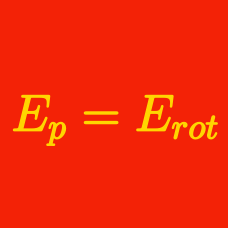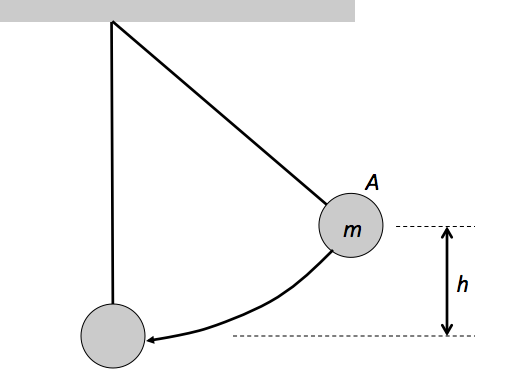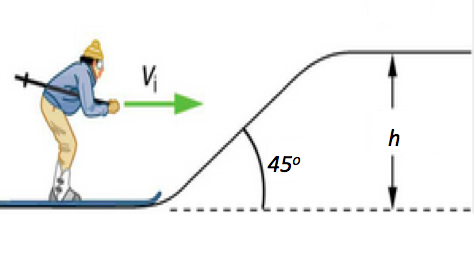Classical Mechanics

# Mixed Conservative and Non-Conservative Forces - Problem Solving

Consider the situation where a baseball player with mass $m = 76 \text{ kg}$ slides to a stop on level ground. Using energy considerations, calculate the distance $d$ the baseball player slides, given that his initial speed is $v = 8 \text{ m/s}$ and the force of friction against him is a constant $f= 304 \text{ N}.$In the above diagram, the bob $A$ of a pendulum with mass $m = 8 \text{ kg}$ is released from height $h = 8 \text{ m}.$ Because of air resistance, the speed of velocity at the lowest position is $v = 6 \text{ m/s}.$ Find the loss in the total mechanical energy of the bob.

The gravitational acceleration is $g =10 \text{ m/s}^2.$

A roller-coaster of mass $M= 2000 \text{ kg}$ has a speed of $v_1 = 10 \text{ m/s}$ as it goes over the top of a $15$-meter-high hill. Then it goes over a another hill of height $10$ meters, and at the crest of that hill, the roller-coaster is moving at $v_2 = 13 \text{ m/s}.$ How much work did friction do on the coaster during its movement between the two hills?

The gravitational acceleration is $g= 10 \text{ m/s}^2.$A skier with mass $m = 77 \text{ kg}$ coasts up an inclination with angle $\theta = 45 ^\circ$ and height $h = 0.5 \text{ m}$ at an initial speed of $v_i = 12\text{ m/s}.$ Find the square of her final speed at the top, given that the coefficient of kinetic friction between her skis and the snow is $\mu = 0.5.$

The gravitational acceleration is $g = 10 \text{ m/s}^2.$

An object with mass $m = 1 \text{ kg}$ at the top of a $h = 7 \text{ m}$ tall hill starts to go down from rest. The downhill portion of the run is covered with frictionless material but the flat section at the base of the hill has a spot of friction $2 \text{ m}$ in length. The object comes straight down the hill, over the spot of friction at the bottom, hits and presses the the damping spring $20 \text{ cm}$ in the process. If the spring constant is $k = 2 \times 10^2 \text{ N/m},$ what is the magnitude of kinetic friction between the object and flat section.

The gravitational acceleration is $g = 10 \text{ m/s}^2$ and air resistance is negligible.

×